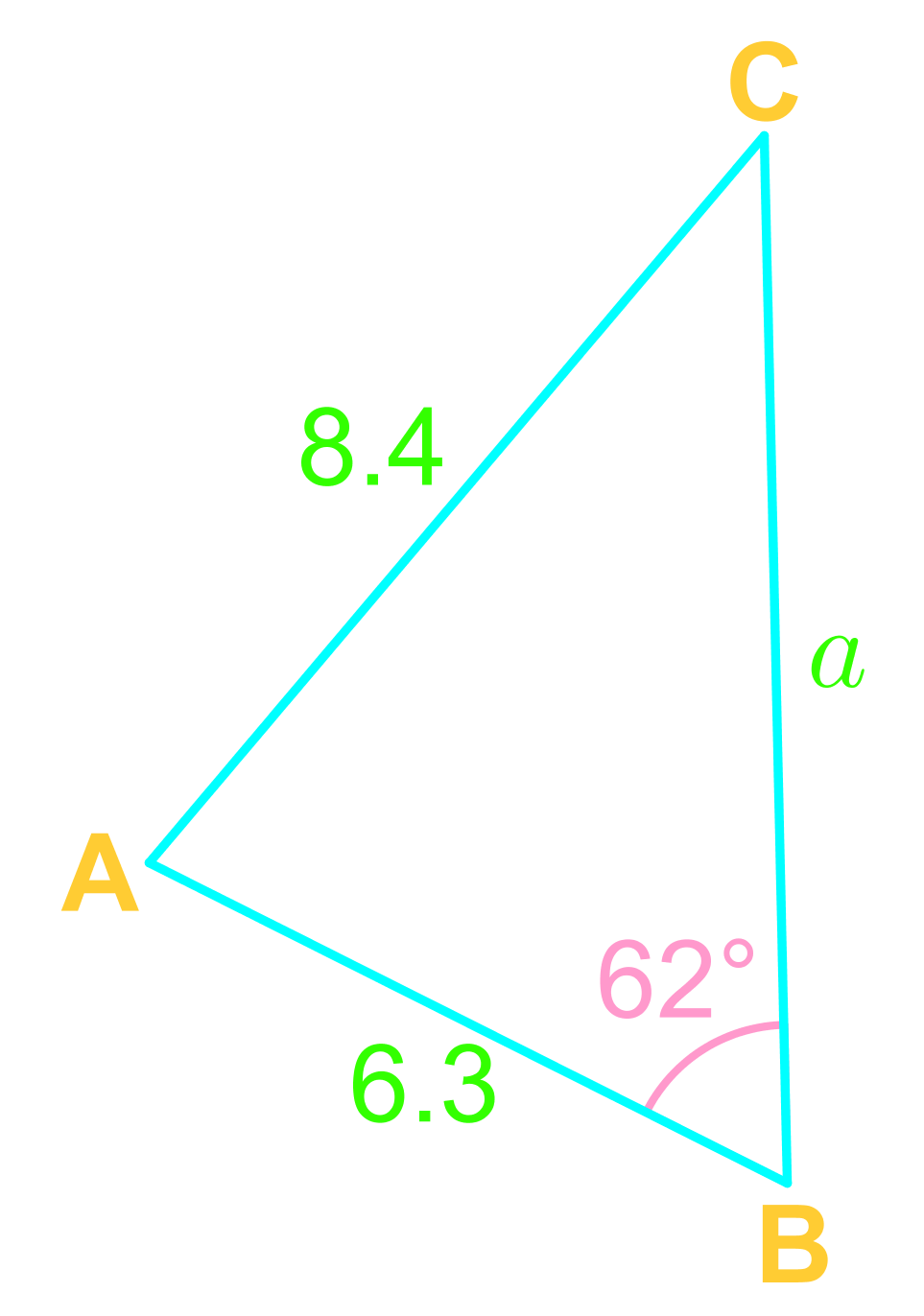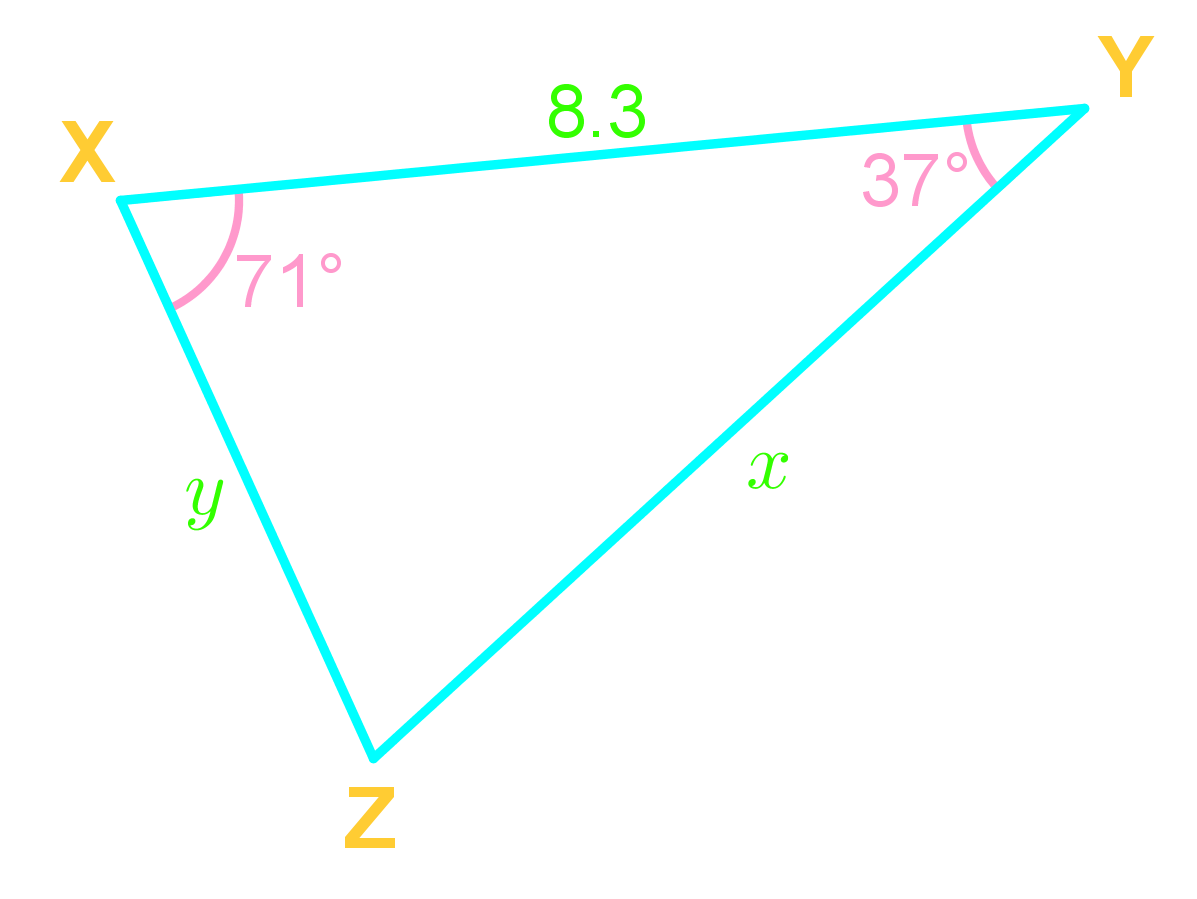# Law of sines

0/1
0/4
##### Examples
###### Lessons
1. Given the following triangle $\triangle ABC$,1. Solve for$\angle C$
2. Solve for $a$
2. Solve for side $x$1. Ambiguous case: SSA triangles
In $\triangle DEF$, $DE=21cm$, $\angle$$F=45$°, and $EF=24cm$; find $DF$.
###### Free to Join!
StudyPug is a learning help platform covering math and science from grade 4 all the way to second year university. Our video tutorials, unlimited practice problems, and step-by-step explanations provide you or your child with all the help you need to master concepts. On top of that, it's fun - with achievements, customizable avatars, and awards to keep you motivated.
• #### Easily See Your ProgressWe track the progress you've made on a topic so you know what you've done. From the course view you can easily see what topics have what and the progress you've made on them. Fill the rings to completely master that section or mouse over the icon to see more details.
• #### Make Use of Our Learning Aids###### Practice Accuracy

See how well your practice sessions are going over time.

Stay on track with our daily recommendations.

• #### Earn Achievements as You LearnMake the most of your time as you use StudyPug to help you achieve your goals. Earn fun little badges the more you watch, practice, and use our service.
• #### Create and Customize Your AvatarPlay with our fun little avatar builder to create and customize your own avatar on StudyPug. Choose your face, eye colour, hair colour and style, and background. Unlock more options the more you use StudyPug.
###### Topic Notes
In this section, we will learn about the Law of Sines, also known as the Sines Rule. The Law of Sines is a formula that models the relationship between the sides and the angles of any triangle, be it a right-angled triangle, an obtuse triangle, or an acute triangle. In order to use the Law of Sines, we need to satisfy the "one pair, one additional information" condition (i.e. Angle-Angle-Side abbreviated as AAS, and Angle-Side-Angle abbreviated as ASA). We will also explore the concept of the Ambiguous Case of the Law of Sines.
Law of Sine
For any $\triangle$ ABC,

$\frac{a}{\sin(A)}$ $=\frac{b}{\sin(B)}$ $=\frac{c}{\sin(C)}$
and,
$\frac{\sin(A)}{a}$ $=\frac{\sin(B)}{b}$ $=\frac{\sin(C)}{c}$

Use the Law of Sine when given a pair!

Ambiguous case
Ambiguous case of the Law of Sine arises when given SSA (side-side-angle)

Step 1) Use the given angle to find the height of the triangle: $h=b \sin (A)$

Step 2) Check if,
$Side\;a$ < $h$,
then no triangles
$Side\;a=h$,
then 1 triangle
$Side\;a$ > $h$,
then 1 triangle
$h$ < $Side\;a$ < $Side\;b$,
then 2 triangles

Step 3) Solve the triangle(s)!